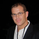9864 görüntülenme
CM_Gap_Indicator_Intra-Day_V2.1

Updated Intra-Day Gap Indicator.

Version 2.1…Many Additional Features To Come.

***Designed for Intra-Day Charts.
***Great for Stocks…Forex and Futures Sunday Night Gaps.

***Plots Green Lines If Gap Up (See Added Features Below)
***Plots Red Lines If Gap Down

***Added Check Boxes In Inputs Tab That Let’s You Choose if You Want To See All Gaps, Or Only Gaps That Open Above/Below Previous Days High/Low

***Gap Mid Lines
***Color Fill Based On Gap Up Or Down

//Created By ChrisMoody on 1-15-2015
//Version 2...added ability to Choose if Gap Up or Down Plots based on Open Being Above/Below Close OR Open Being Above/Below Previous Days High/Low

study(title="CM_Gap_Indicator_Intra-Day_V2.1", shorttitle="CM_Gaps_Intra-Day_V2.1", overlay=true)

guc = input(true, title="Gap Up Criteria - IF CHECKED = Open is > Than Previous Days CLOSE, If NOT CHECKED = Open is > than Previous Days HIGH")
gdc = input(true, title="Gap Down Criteria - IF CHECKED = Open is < Than Previous Days CLOSE, If NOT CHECKED = Open is < than Previous Days LOW")

//Criteria using Daily Sessions
tdo = security(tickerid, 'D', open)
pdc = security(tickerid, 'D', close)
pdh = security(tickerid, 'D', high)
pdl = security(tickerid, 'D', low)

midLine = abs((pdc + tdo)*.5)

gapUpPct = guc and tdo > pdc ? tdo > pdc : tdo > pdh

plotGapUpLine = gapUpPct == 1 ? tdo : na
plotGapUpLowLine = gapUpPct == 1 ? pdc : na
plotGapUpMidLine = gapUpPct == 1 ? midLine : na

gapDnPct = gdc and tdo < pdc ? tdo < pdc : tdo < pdl

plotGapDnLine = gapDnPct == 1 ? tdo : na
plotGapDnUpLine = gapDnPct == 1  ? pdc : na
plotGapDnMidLine = gapDnPct == 1 ? midLine : na

p1 = plot(plotGapUpLine, title="Gap Up - Upper Gap Line, Todays Open", style=circles, linewidth=3, color=lime)
p2 = plot(plotGapUpLowLine, title="Gap Up - Lower Gap Line, Yesterdays Close", style=circles, linewidth=3, color=lime)
plot(plotGapUpMidLine, title="Gap Up - Mid Line", style=circles, linewidth=2, color=gray)
fill(p1, p2, color=green, transp=70)

p3 = plot(plotGapDnLine, title="Gap Down - Lower Gap Line, Todays Open", style=circles, linewidth=3, color=red)
p4 = plot(plotGapDnUpLine, title="Gap Down - Upper Gap Line, Yesterdays Close", style=circles, linewidth=3, color=red)
plot(plotGapDnMidLine,  title="Gap Down - Mid Line", style=circles, linewidth=2, color=gray )
fill(p3, p4, color=red, transp=85)

## Yorumlarelp
No Problem...more features to come soon
Cevap Gönder